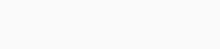#### What is Discrete Distribution?

In statistics, a discrete distribution is a distribution with discrete values. Numerical values, such as 1, 10, 15, etc., can be counted as discrete, finite, non-negative numbers. Its is One of the foundation of statistical analysis and probability theory and As previously mentioned, discrete distributions are those whose values are countable whole numbers.

One of the example of discrete probability distribution is Poisson distribution which is covered earlier.

#### Understanding Discrete Distribution

Data distributions are statistical concepts used in data analysis. A probability distribution diagram represents the distribution of measurable data points from a data set in order to determine the outcomes and probabilities of a particular study. The normal distribution (“bell curve”) is one type of probability distribution diagram that can be derived from a distribution study.

Depending on the type of outcome to be measured, statisticians can identify whether a discrete or continuous distribution will develop. Unlike the normal distribution, which is continuous and can account for any possible outcome along the number line, the discrete distribution is constructed from data that can only be followed by a finite or discrete set of outcomes

A discrete random variable takes values confined to a range of separate or ‘discrete’ values. (More formally, a discrete random variable takes either a finite number of values or a countably infinite number of values.)

In the example, the first two random variables Ui and Vi are counts: they can only take non-negative integer values. (A person cannot have moved residence 2.3 times by age 18, nor can a person have owned 9.6 mobile phones.) A random variable based on a count is an example of a discrete random variable.

Discrete distributions represent data with a countable number of outcomes, which means the possible outcomes can be listed. The list can be finite or infinite.

Monte Carlo simulations can also reveal discrete distributions. The Monte Carlo simulation is a mathematical model that identifies the probabilities of different outcomes by using a computer program. Generally, it is used to forecast scenarios and identify risks. Monte Carlo simulation produces discrete distributions for analysis of outcomes with discrete values. To determine risk and trade-offs among various items being considered, these distributions are used.

In contrast, continuous distributions are characterized by outcomes that may fall anywhere along a continuum.

Distributions such as these are often based on statistical analyses of “counts” or “how often” an event occurs.

In finance, discrete distributions are used to price options and forecast market stocks or recessions.A graph of discrete distribution looks like this which represents which part of sample set has what volume.

Lets consider an example of calculating height of candidates for police recruitment procedure. We can’t have something which is negative so here discrete distribution is ideal for use as it has all the property which required like discrete, finite, non-negative numbers. To analyze and for stats here it will let you now of numbers of candidates having heights with respect to region, gene etc which can be very useful.

#### Variance

The variance of a discrete random variable X can be calculated as follows: var(X) = X (x ) 2 pX (x),

The sum is taken over all values of x for which pX (x) > 0. The variance of X is the weighted average of the squared deviations from the mean , where the weights are determined by the probability function pX (x) of X.

#### Mean

In discrete random variables X, the mean X is given by X = X x pX (x), where X represents all x values where pX (x) > 0.

#### coding part

Below we will see that how a discrete distribution works in simple terms.

As you can see we have a function which carries out the defined arrays of int with some of its property defined.

				
// it is a descrete function which will demonstrate us the dicrete probability distribution
fun discretedistribution(args: Array) // defining the arrays of type int
{
val a = args.size
var totalsize = 0



With the condition mentioned below you can see that we are trying to get the array and generate it for further use.

				
// condition for arrays
for (i in 0 until a)
{
totalsize += args[i]
}
// declared to generate random variable each time for distribution
val r = (totalsize * Math.random()).toInt() // for discrete probability distributon



Here in this block we have values which are entered with respect to the discrete probability and printing the values of that event.

				
// manually enterd values for the array
var sumof = 0
var eventof = -1
var i = 0
// condition for probability
while (i &lt; a &amp;&amp; sumof &lt;= r)
{
sumof += args[i]
eventof = i
i++
}
println(eventof)
}



Finally we are representing the value with function created earlier.

				
// final for printing the value from arrays in order to display descrete probability distribution
val b = arrayOf(1, 2, 3, 4, 5)
discretedistribution(b)



#### OutputHere we conclude for this topic hope you have learned something new of discrete probability distribution.